# Place of a field

(diff) ← Older revision | Latest revision (diff) | Newer revision → (diff)with values in a field,-valued place of a fieldA mapping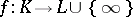satisfying the conditions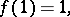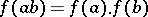(provided that the expressions on the right-hand sides are defined). The following conventions are made: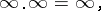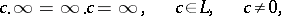while the expressions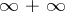,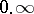and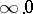are undefined.

An elementinfor which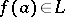is called finite in the place; the setof finite elements is a subring of, and the mappingis a ring homomorphism. The ringis a local ring, its maximal ideal is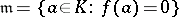.

A placedetermines a valuationofwith group of values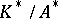(where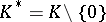and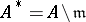are, respectively, the groups of invertible elements ofand). The ring of this valuation is the same as. Conversely, any valuationof a fielddetermines a place ofwith values in the residue class field of. Here, the ring of finite elements is the same as the ring of (integers of) the valuation.

How to Cite This Entry:
Place of a field. Encyclopedia of Mathematics. URL: http://encyclopediaofmath.org/index.php?title=Place_of_a_field&oldid=17112
This article was adapted from an original article by Yu.G. Zarkhin (originator), which appeared in Encyclopedia of Mathematics - ISBN 1402006098. See original article